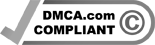Earlier today I set you the following three problems, taken from this year’s Mathigon puzzle advent calendar.

1. The seven digits

Solution: 2

Here’s one way to arrange the numbers:

First, we can eliminate the digits 0, 5 and 7 from the solution. If 0 was used once, then at least one of the products would be zero, but at least one would be nonzero, a contradiction. If 5 was used once, one of the products would be a multiple of 5, but since no other digits are a multiple of 5, there would also be a product that was not a multiple of 5, again a contradiction. Likewise with 7.

At least one of the products contains 8, and at least one of the products contains 9 as a factor. Since these two numbers are co-prime (meaning they dont share any prime factors) the product needs to be at least 8 × 9 = 72. If you haven’t come across (or forgot) the idea of co-primality, you would by a process of trial and error come to the conclusion that the products had to be at least 72.

If we rewrite the digits in terms of their prime factors we have: 1, 2, 3, 2×2, 2×3, 2x2x2, 3×3. There are four 3s and seven 2s. Since we have to split the number of 2s and 3s evenly between the left product and the right product, the middle digit has to be 2.

2. A plurality of paths.

Solution: 70

The least technical way of solving this puzzle is to count how many ways there are to get to every intersection in the grid, starting in the top-left corner. Along the first row and column, there is only a single way to get to each intersection: either going straight to the right, or straight down. For all other intersections, the number is simply the sum of the two previous intersections, going up or to the left. If we repeat this for the entire grid, we also get 70 as the answer.

But wait! The numbers in the intersections are one of the most famous and useful number patterns in maths: Pascal’s triangle. (To find out more, here is Mathigon’s page on Pascal’s triangle.)

3. Flipping frogs.

Solution: yes

First, let’s just try to swap the four frogs in the middle row. This is possible in 8 steps, and below is one possible solution. Notice that every square in the middle row is empty at some point in this process. For example, the middle square is empty to start with, and the last square is empty after the third step.

Now we insert some additional steps into our solution. Whenever a square in the middle row is empty, we use that opportunity to swap the four frogs in that column – using the same steps used to swap the frogs in the middle row. Once we’ve swapped both the middle row and each of the five columns, the solution is complete. Ta-dah! Or should that be Toad-aha!

I hope you enjoyed these puzzles. I’ll be back in two week.s

Thanks to Mathigon for today’s puzzles. They come from the Mathigon puzzle advent calendar, which starts on Wednesday December 1.

I set a puzzle here every two weeks on a Monday. I’m always on the look-out for great puzzles. If you would like to suggest one, email me.

I also give school talks about maths and puzzles (restrictions allowing). If your school is interested please get in touch.

I’m the author of several books of maths and puzzles , most recently The Language Lover’s Puzzle Book, but all of which would make fantastic Christmas gifts!